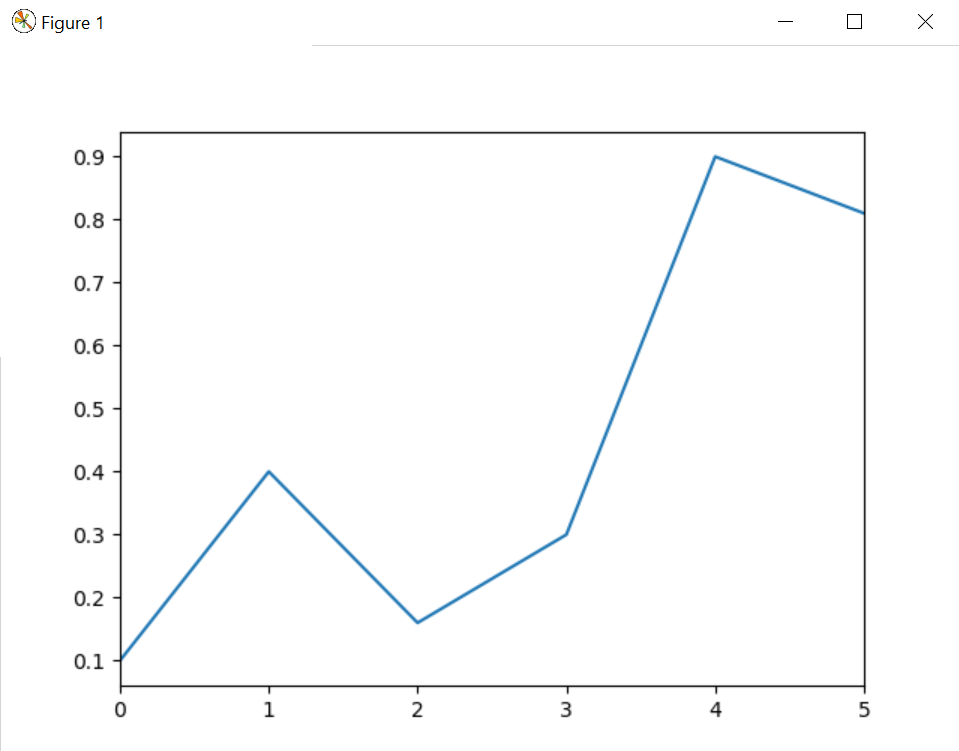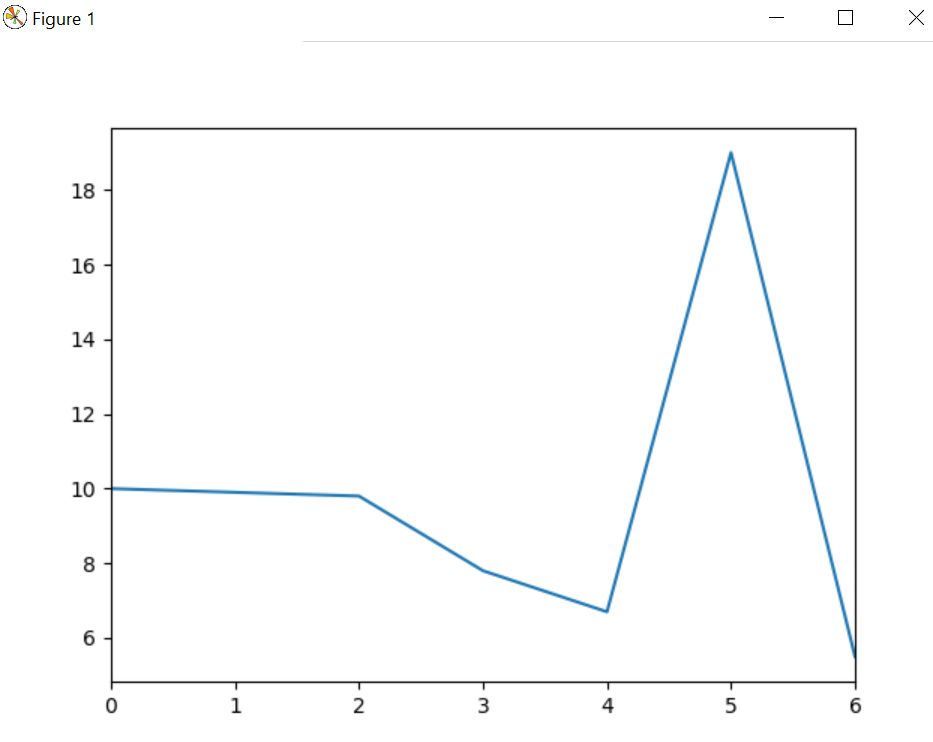# Python | Pandas Series.plot() method

• Difficulty Level : Medium
• Last Updated : 17 Sep, 2019

With the help of `Series.plot()` method, we can get the plot of pandas series by using `Series.plot()` method.

Syntax : `Series.plot()`
Return : Return the plot of series.

Example #1 :
In this example we can see that by using `Series.plot()` method, we are able to get the plot of pandas series.

 `# import Series and matplotlib``import` `pandas as pd``import` `matplotlib.pyplot as plt`` ` `# using Series.plot() method``gfg ``=` `pd.Series([``0.1``, ``0.4``, ``0.16``, ``0.3``, ``0.9``, ``0.81``])`` ` `gfg.plot()``plt.show()`

Output :Example #2 :

 `# import Series and matplotlib``import` `pandas as pd``import` `matplotlib.pyplot as plt`` ` `# using Series.plot() method``gfg ``=` `pd.Series([``10``, ``9.9``, ``9.8``, ``7.8``, ``6.7``, ``19``, ``5.5``])`` ` `gfg.plot()``plt.show()`

Output :My Personal Notes arrow_drop_up